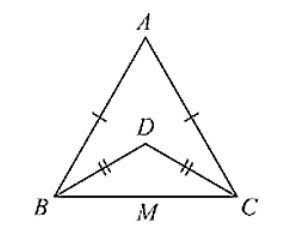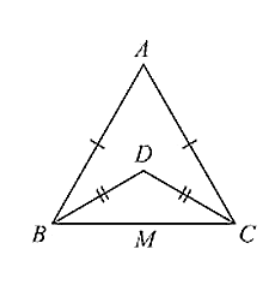# In Fig. (10).2(5) AB = AC and DB = DC, find the ratio ∠ABD: ∠ACD.

Question:

In Fig. (10).2(5) AB = AC and DB = DC, find the ratio ∠ABD: ∠ACD.Solution:

Consider the figure

Given,

AB = AC, DB = DC and given to find the ratio

∠ABD = ∠ACD

Now, ΔABC and ΔDBC are isosceles triangles since AB = AC and DB = DC respectively

∠ABC = ∠ACB and ∠DBC = ∠DCB [Angles opposite to equal sides are equal]

Now consider,

∠ABD : ∠ACD

(∠ABC - ∠DBC): (∠ACB - ∠DCB)

(∠ABC - ∠DBC): (∠ABC - ∠DBC) [∠ABC = ∠ACB and ∠DBC = ∠DCB]

1: 1

ABD: ACD = 1: 1